#### How to Find Factorial in Excel

Factorials are widely used in Mathematical analysis. It has a simple calculation in Mathematical perspective and can be applied to real-world phenomena as well. However, factorial calculations can be executed with a simple built-in function in Excel, which is pretty straightforward.

This is an ultimate guide to cover how to find factorial in Excel and its different applications. The whole content in this Excel tutorial has been summed up into the following outline. Jump into the desired title by skipping irrelevant titles.

## What is factorial?

Understanding what’s factorial is not as hard as it sounds for you. Because it’s a simple calculation as long as you apply it accurately to any given scenario.

Factorial determines the number of orders or combinations that can be arranged for a given set of items. It is mathematically denoted by the exclamation mark (!). In other words, the factorial of a number is the multiplication of each whole number from the chosen number to 1.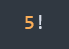For an example, the factorial of 5 is represented mathematically as follows.

Thus, factorial can be denoted for a given number (n).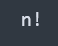## Why is factorial used?

Besides the mathematical definition of factorial, there are applications for real-world phenomena where factorial is used.

The most common applications of factorial are combinations and permutations. But you don’t have to know what they are to use factorial.

Imagine that you have to determine the possible number of combinations that you can make from 4 different colors. You may have a hard time creating those combinations one by one to determine the total number as the final count.

Thanks to factorial and multiplication, you can calculate the total number of combinations by factorial 4 (!4) easily. Keep reading!

## Calculation of factorial

Calculating the factorial for a given number is simply done by multiplying a set of numbers that are determined from the given number.

For an example, the factorial of five is the product of all integers which are less than or equal to 5. It can be simply represented as follows.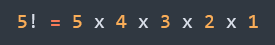The factorial 5 is equal to 120 and can be mathematically represented as follows.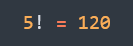So, for a given n items, the factorial n (! n) is calculated.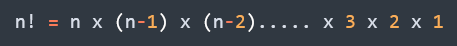### Factorial of a negative number

You might wonder what could be the factorial of a negative number. Factorial of any given negative integer is undefined.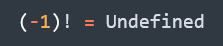### Factorial of a decimal number

The factorial of a decimal number can be determined by using Gamma Function which is out of scope of this article. However, factorials of negative decimal places do exist but not negative integers.

### Factorial of 0 (0!)

Can you figure it out? What could be the factorial of zero being equal to? 0! = 1 by common convention. Definitely it looks odd, but there are explanations in Math to justify this expression.

### Factorial of 1 (1!)

It is easy to determine the factorial(1) by its own definition. Number of arrangements that can be done from a one item for a given set is 1. Thus 1! = 1.

## Factorial in Excel

If you ever expect that the same mathematical notation of factorial works in Excel, no it doesn’t. Excel provides a built-in function, FACT to find a factorial for any given number.

Syntax

=FACT(number)

Arguments

FACT function has only one argument, number which is required. It is the number for which the factorial that you want to calculate and should be non-negative. If the number has decimal points, they’d be truncated.

## How to find factorial in Excel?

Firstly, prepare your spreadsheet along with parameters that need to do calculation of factorial. Click on any cell in your spreadsheet where you have to retrieve the result of factorial.

Now include the required syntax of factorial in the cell.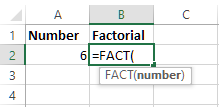Or

Use the Formula bar on top of the spreadsheet to include the required syntax of factorial.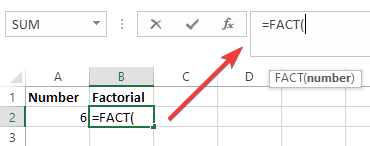Now you have to provide the number as argument for which you need to have the factorial calculated. In Excel, the argument can be a hard-coded number or a cell address.

So, we need to insert the cell address for this example as we have the number in a different cell in the spreadsheet.

To do that, click on the cell which includes the number. So, the address of the selected cell will be visible as the argument of the Fact function.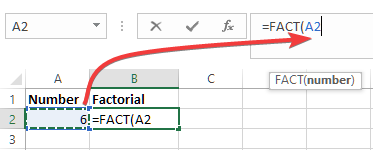Click ‘Enter’ to retrieve the result on the desired cell.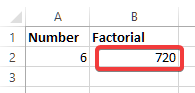You might need to revise or change the parameters of the formula that you just created. If you want to revise the formula which determined the result, double click within the cell that includes the result.It will highlight the formula and the cells of parameters as illustrated in the above image.

### Examples

Here are some examples to indicate how factorial varies depending on the type of number in Excel. You will note certain limitations or differences against the original definitions of some types of numbers in Mathematics.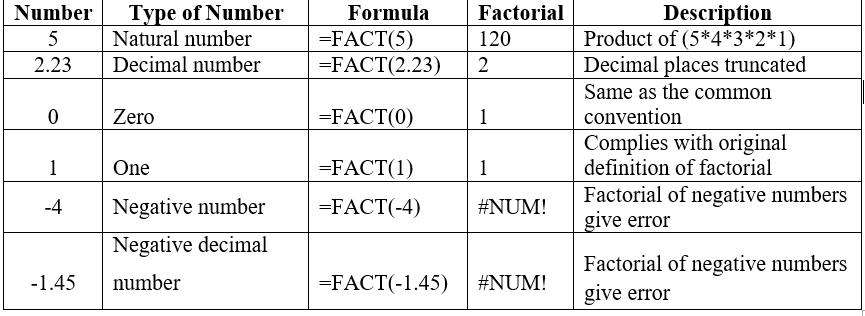## VBA code

You can find the factorial of a number in Excel VBA using a recursive function. Here’s an example:

`Function Factorial(n As Long) As Double `

` If n <= 1 Then `

`  Factorial = 1 `

` Else `

`  Factorial = n * Factorial(n - 1) `

` End If `

`End Function`

This function takes an argument n and calculates the factorial of n using recursion. If the value of n is less than or equal to 1, the function returns 1. If n is greater than 1, the function calls itself with n-1 and multiplies the result by n.

You can call this function in your VBA code or in a cell in your spreadsheet. For example:

=Factorial(5)

This would return 120 as the factorial of 5 is 5 x 4 x 3 x 2 x 1 = 120.

## Different applications of factorial in Excel

Let’s find out how it’s easy to convert a mathematical formula to an Excel formula. These mathematical formulas are examples of permutations and combinations where factorials are used for the calculations.

### Permutations

Permutations are the number of possible ways that a given set of items can be ordered or arranged. So, we do careful about the order or arrangement of the items in the set. Let’s quickly go through the following example to calculate it in Excel.

Example

A product code is issued for a set of eBooks where first 3 letters are a combination of A, B, C, D and E. No letter is repeated more than once in a particular code. You are required to determine how many eBooks can be labeled with a given unique code.

Solution – Reasoning

You can make 5 possible choices out of 5 letters for the first character of the code. Once you chose a letter, you are left with 4 possible choices for the second character of the code. As the final choice, you are left with 3 possible choices for the third character of the code.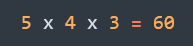There are 60 eBooks that you can prepare with unique product codes.

Solution – Permutation Function

When you have to create combinations and order matters from a given set of items, that’s where permutation comes in. So, you can easily apply the permutation function to determine the result.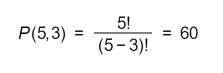Solution – Factorial in Excel

You can simply use FACT function in Excel to determine the answer for the above example. Parameters are not cell addresses as you can note just to simply the calculation for illustration purposes.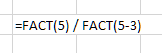### Combinations

Combinations are the number of possible ways that a given set of items can be arranged where order of the arrangement does not matter. For an instance you are probably required to make a selection out of a given set of items regardless of the order of the selection.

Let’s quickly go through the following example to calculate it in Excel.

Example

Suppose you are going to have carrom matches with 5 friends including you. 4 players will play in each game. Now you need to know how many games can be played where each one of you will play with every one else in the group.

Solution – Combination Function

4 players should be selected at a time from the group for a match. The number of matches can be easily determined using the combination function.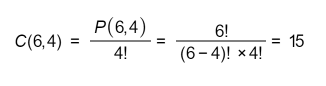Solution – Factorial in Excel

You can just replace the FACT function in Excel instead of factorials in above equation. Parameters are not cell addresses as you can note just to simply the calculation for illustration purposes.

Make sure to use parentheses correctly as shown above. Otherwise, the accurate result will not show in Excel.

Doesn’t it make you feel uncomfortable to struggle with paratheses as shown in the above equation in Excel spreadsheet? Excel has made it ease to yield the results from combinations from another particular function which is specific to it.

You can simply use COMBIN function for the above example as shown below.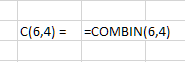So, you can have the result same as the above formula where factorials are used.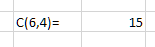Note: You can download the Excel file which includes all the examples explained in this tutorial by clicking here.